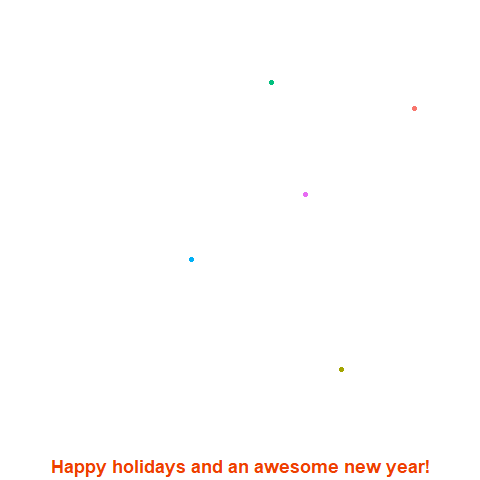# R fireworks!

Happy holidays! Happy new year! As a last project and seasonal greetings I wanted to simulate fireworks within R With `gganimate`. And it really worked! See the awesome result:Basically the code generates a lot of points and gets different samples. It’s then using the Kmeans algorithm to get the clusters of points and with that the colors. The amount of clusters is randomized between 1 and 10. This is repeated a couple of times. And these repetitions are also the different times for `transition_time`. I added a bit of randomness between the clusters within the times. So the fireworks go off staggered within each time section.

It’s fun project and there are still a couple of ways to enhance this animation. One thing I wanted to add were drawing the lines from the center to the end points. I tried `transition_reveal` with `geom_line`. The animation covered only half of the lines each cluster. So maybe someone else can make that work. Another thing is the beginning and end of the animation are a bit awkward. The beginning always seem to shoot all the fireworks for that time frame at once and the end seems to cut off the last few animations.

Here is the code to make your own fireworks. Have fun! Happy new year!

``````library(tidyverse)
library(gganimate)

# make a df
df <- data.frame(x = runif(100000),
y= runif(100000))

## get the outer limits for the plot
xmax <- max(df\$x)
xmin <- min(df\$x)
ymax <- max(df\$y)
ymin <- min(df\$y)

## make a loop for n firework displays. Is that the term?
fireworks <- list()
mean_t <- 1
begin_gr <- 400

for (i in 1:20){

mean_t <- mean_t + 2

## random amount of clusters 1 - 10
nclust <- runif(1, min = 1, max = 10)

## random time for fireworks + some wait time from last display

t <- data_frame(t = round(rnorm(n = nclust, mean = mean_t, sd = 0.3), 1),
cl = 1:nclust)

df_s <- data.frame(x = sample(df\$x, 150),
y = sample(df\$y, 150))

## all points to diferent groups for the animation
begin_gr <- begin_gr + nrow(df_s) + 1
end_gr <- begin_gr + nrow(df_s) - 1

km <- kmeans(df_s, nclust, iter.max = 50)

cluster_info <- data.frame(cl = km\$cluster)

fw  <-  df_s %>%
bind_cols(cluster_info) %>%
arrange(cl) %>%
mutate(gr = begin_gr:end_gr)

df_cl <- fw %>%
group_by(cl) %>%
summarise(nr = n())

ntimes <- df_cl\$nr

## starting position from centroids of the clusters
centre_fw <- data.frame(x = rep(km\$centers[,1], ntimes),
y = rep(km\$centers[,2], ntimes),
cl = rep(1:nrow(km\$centers), ntimes),
gr = begin_gr:end_gr)

fireworks[[i]] <- fw %>%
left_join(t, by = "cl") %>%
## add starting position with t - 0.5
bind_rows(centre_fw %>% left_join(t, by = "cl") %>% mutate(t = t-0.5)) %>%
mutate(cl = as.factor(cl))
}

fireworks <- bind_rows(fireworks)

ani <-fireworks %>%
ggplot(aes(x = x, y = y)) +
geom_point(data = fireworks %>% filter(cl == 1), aes(group = gr, col = cl), size = 2) +
geom_point(data = fireworks %>% filter(cl == 2), aes(group = gr, col = cl), size = 2) +
geom_point(data = fireworks %>% filter(cl == 3), aes(group = gr, col = cl), size = 2) +
geom_point(data = fireworks %>% filter(cl == 4), aes(group = gr, col = cl), size = 2) +
geom_point(data = fireworks %>% filter(cl == 5), aes(group = gr, col = cl), size = 2) +
## turn of everything manually, theme_void() won't cooperate with my happy holidays msg
xlab( "Happy holidays and an awesome new year!") +
theme(axis.line = element_blank(),
axis.text.y = element_blank(),
axis.text.x = element_blank(),
axis.ticks = element_blank(),
axis.title.y = element_blank(),
legend.position = "none",
panel.background = element_blank(),
panel.border = element_blank(),
panel.grid.major = element_blank(),
panel.grid.minor = element_blank(),
plot.background = element_blank(),
axis.title.x = element_text(face = "bold", colour = "orangered2", size = 18)) +
scale_x_continuous(limits = c(xmin, xmax)) +
scale_y_continuous(limits = c(ymin, ymax)) +
## gganimate options
transition_time(t) +